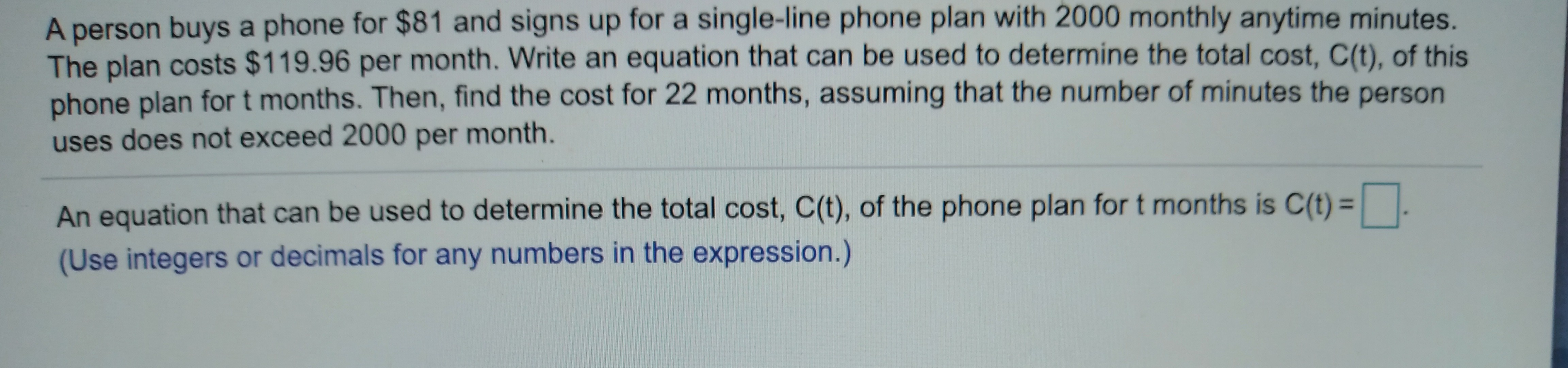# A person buys a phone for \$81 and signs up for a single-line phone plan with 2000 monthly anytime minutes.The plan costs \$119.96 per month. Write an equation that can be used to determine the total cost, C(t), of thisphone plan for t months. Then, find the cost for 22 months, assuming that the number of minutes the personuses does not exceed 2000 per month.An equation that can be used to determine the total cost, C(t), of the phone plan for t months is C(t) || .(Use integers or decimals for any numbers in the expression.)

Question
60 viewshelp_outlineImage TranscriptioncloseA person buys a phone for \$81 and signs up for a single-line phone plan with 2000 monthly anytime minutes. The plan costs \$119.96 per month. Write an equation that can be used to determine the total cost, C(t), of this phone plan for t months. Then, find the cost for 22 months, assuming that the number of minutes the person uses does not exceed 2000 per month. An equation that can be used to determine the total cost, C(t), of the phone plan for t months is C(t) || . (Use integers or decimals for any numbers in the expression.) fullscreen
check_circle

Step 1

Refer to the question,

As person buys a phone at \$81 and monthly plan costs \$ 119.96

Let t be the number of months  and C(t) be the total cost of t months.

Step 2

As the phone cost \$86 which is fixed cost  and monthly plan costs \$ 119.96 is a...

### Want to see the full answer?

See Solution

#### Want to see this answer and more?

Solutions are written by subject experts who are available 24/7. Questions are typically answered within 1 hour.*

See Solution
*Response times may vary by subject and question.
Tagged in

### Algebra